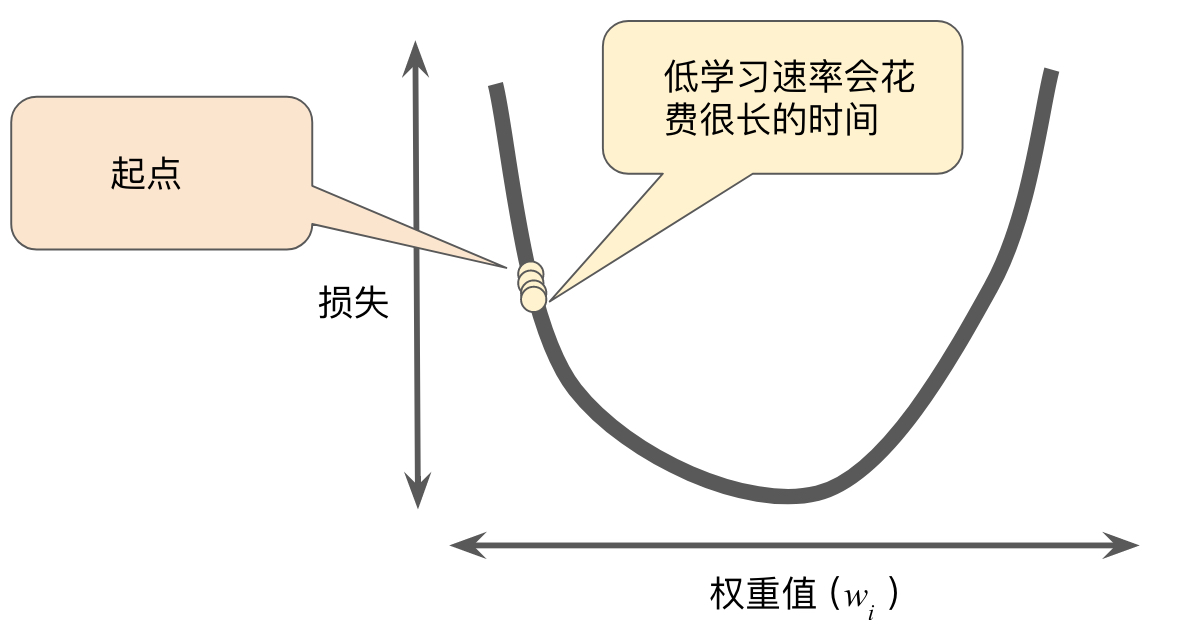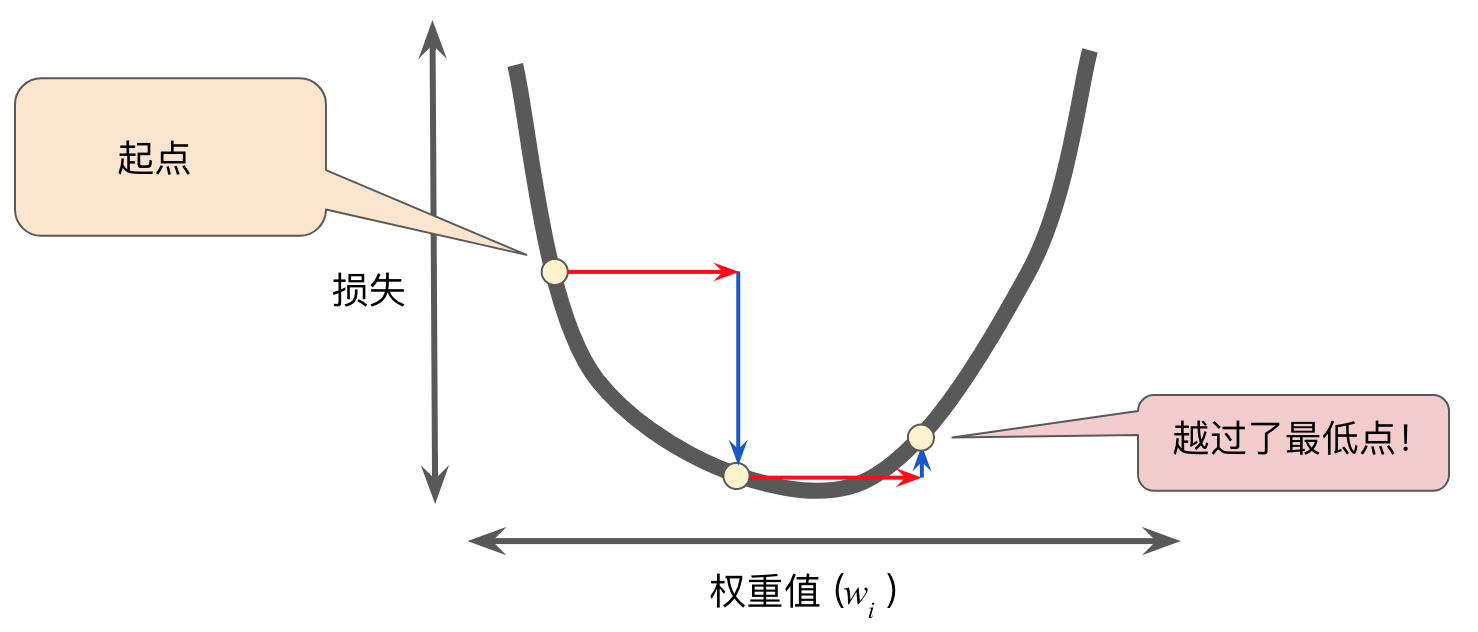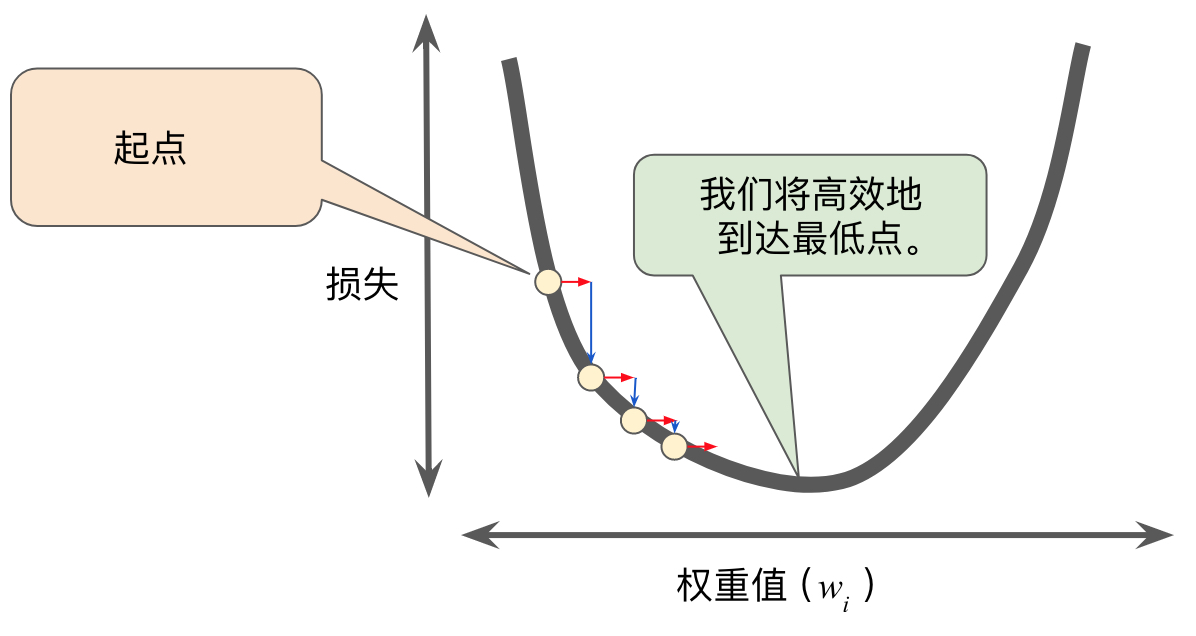## 降低损失

### 迭代方法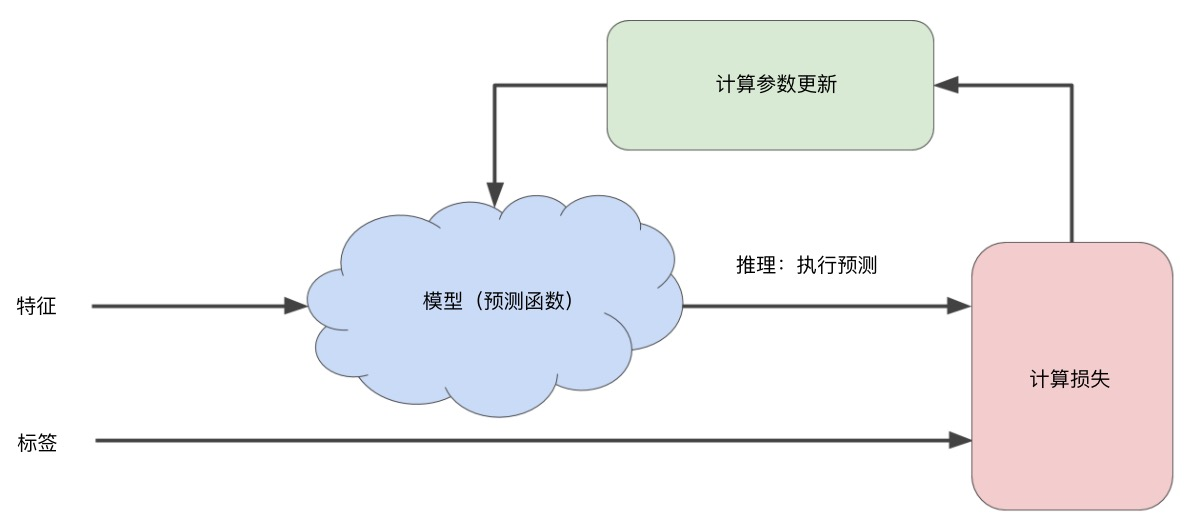“模型”部分将一个或多个特征作为输入，然后返回一个预测 $y^{‘}$ 作为输出。为了进行简化，不妨考虑一种采用一个特征并返回一个预测的模型：

$$y^{‘}=b+w_{1}x_{1}$$

• $b=0$
• $w_{1}=0$

$y^{‘}=0+0(10) \\ y^{‘}=0$

• $y^{‘}$，模型对特征 $x$ 的预测
• $y$，特征 $x$ 对应的正确标签

### 梯度下降法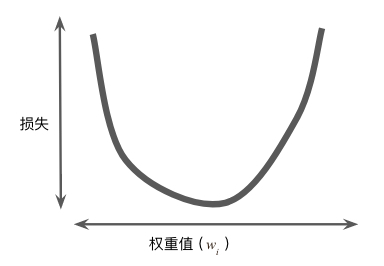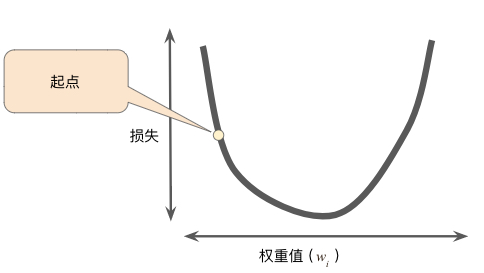#### 导数和梯度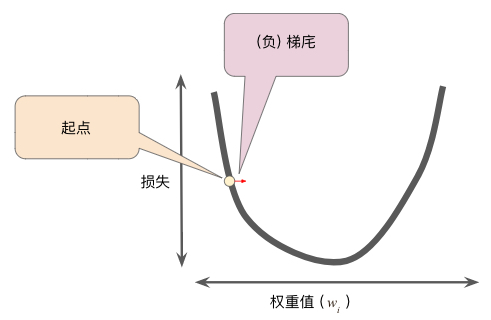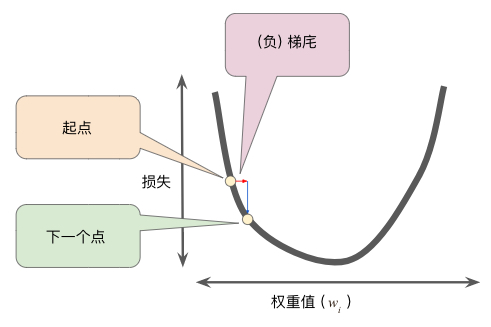### 学习速率